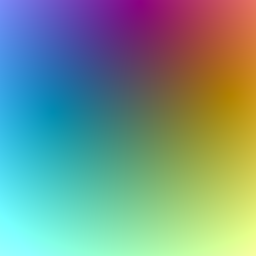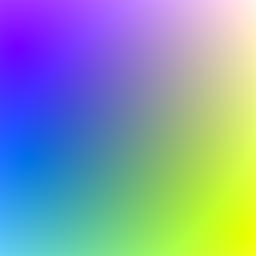example processing for-loop
``````float redX;
float redY;
float redXSpeed;
float redYSpeed;

float greenX;
float greenY;
float greenXSpeed;
float greenYSpeed;

float blueX;
float blueY;
float blueXSpeed;
float blueYSpeed;

void setup() {
size(256, 256);
noSmooth();

redX = random(width);
redY = random(height);
redXSpeed = random(-1, 1);
redYSpeed = random(-1, 1);

greenX = random(width);
greenY = random(height);
greenXSpeed = random(-1, 1);
greenYSpeed = random(-1, 1);

blueX = random(width);
blueY = random(height);
blueXSpeed = random(-1, 1);
blueYSpeed = random(-1, 1);
}

void draw() {

redX += redXSpeed;
redY += redYSpeed;
if (redX < 0 || redX > width) {
redXSpeed *= -1;
}
if (redY < 0 || redY > height) {
redYSpeed *= -1;
}

greenX += greenXSpeed;
greenY += greenYSpeed;
if (greenX < 0 || greenX > width) {
greenXSpeed *= -1;
}
if (greenY < 0 || greenY > height) {
greenYSpeed *= -1;
}

blueX += blueXSpeed;
blueY += blueYSpeed;
if (blueX < 0 || blueX > width) {
blueXSpeed *= -1;
}
if (blueY < 0 || blueY > height) {
blueYSpeed *= -1;
}

for (int y = 0; y < height; y++) {
for (int x = 0; x < width; x++) {
float redDistance = dist(x, y, redX, redY);
float greenDistance = dist(x, y, greenX, greenY);
float blueDistance = dist(x, y, blueX, blueY);

stroke(redDistance, greenDistance, blueDistance);
point(x, y);
}
}
}
``````
Code Editor

See the Pen by Happy Coding (@KevinWorkman) on CodePen.

This code consists of two main parts: it has three points that bounce around the screen, and it uses nested `for` loops to loop over every pixel in the window. For each pixel, it creates a color based on that pixel’s distance from the three bouncing points, and then draws the pixel in that color. This creates a gradient that transitions through different colors as the points bounce around.If this code seems complicated, focus on each part separately. The bouncing balls are covered here, and the radial gradients are covered here.## Tweak Ideas

• Change how the points move around the screen. Make them move in random directions instead of straight lines, or make them turn in circles, or make them wrap around the screen instead of bouncing off the edges.
• Add two more points: a white light source and a black light source. They should feed into the existing parameters.